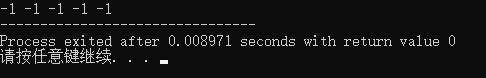• 转载 #include<iostream> #include <vector> int main() { //1. 不带参数的构造函数初始化 vector<... //2.... //初始化了10个默认值为0的元素 vector<int> cde(10, 1); //初

转载

#include<iostream>
#include <vector>

int main()
{
//1. 不带参数的构造函数初始化
vector<int> vec;
cout << vec.size() << endl;

//2. 带参数的构造函数初始化
vector<int> abc(10);    //初始化了10个默认值为0的元素
vector<int> cde(10, 1);    //初始化了10个值为1的元素

//3. 通过数组地址初始化
int a = { 1,2,3,4,5 };
//通过数组a的地址初始化，注意地址是从0到5（左闭右开区间）
vector<int> b(a, a + 5);

//4. 通过同类型的vector初始化
vector<int> c(5, 1);
//通过a初始化
vector<int> d(c);

//5. 通过insert初始化
//insert初始化方式将同类型的迭代器对应的始末区间（左闭右开区间）内的值插入到vector中
vector<int> e(6, 6);
vector<int> f;
//将e~e插入到f中，f.size()由0变为3
f.insert(f.begin(), e.begin(), e.begin() + 3);
//insert也可通过数组地址区间实现插入
int a = { 6,6,6,6,6,6 };
vector<int> b;
//将a的所有元素插入到b中，同样是左闭右开区间
b.insert(b.begin(), a, a + 6);
//此外，insert还可以插入m个值为n的元素
//在b开始位置处插入6个6
b.insert(b.begin(), 6, 6);

//6. 通过copy函数赋值
vector<int> x(5, 1);
int x1 = { 2,2,2,2,2 };
vector<int> y(10);

/*将a中元素全部拷贝到b开始的位置中,注意拷贝的区间为a.begin() ~ a.end()的左闭右开的区间*/
copy(x.begin(), x.end(), y.begin());

//拷贝区间也可以是数组地址构成的区间
copy(x1, x1 + 5, y.begin() + 5);
}

展开全文vector c++
• 给定一个 n × n 的矩阵，求它顺时针旋转 90 度的结果，且必须在原矩阵上修改（in-place）。...vector> using namespace std; class Matrix { public:Matrix(); ~Matrix(); void rotate(vector<vecto

记录直接声明并定义二维数组vector：

vector<vector<string>> tickets = { {"MUC""LHR"}, { "JFK" "MUC" }, { "SFO" "SJC" }, { "LHR" "SFO" } };

vector<pair<int, int>>sortB(length);
for (int i = 0; i < length; i++) {
sortB[i] = { nums2[i], i };
}

给定一个 n × n 的矩阵，求它顺时针旋转 90 度的结果，且必须在原矩阵上修改（in-place）。
怎样能够尽量不创建额外储存空间呢？

`cpp
#pragma once
#ifndef MATRIX_H_INCLUDE
#define MATRIX_H_INCLUDE
#include <vector>
using namespace std;

class Matrix
{
public:Matrix();
~Matrix();
void rotate(vector<vector<int> >& vec);
private:

};

#endif // !MAXT

注释掉的初始化和利用迭代器输出vector二维数组都是可运行的

void main()
{
int n = 3;
int temp;
vector<vector<int> > vec(n);
Matrix matrix;

//初始化vector
//for (int i = 0; i < n; i++)
//{
//	vec[i].resize(n);
//}

//输入
printf("INPUT：[\n");
for (int i = 0; i < n; i++)
{
printf("[");
for (int j = 0; j < n; j++)
{
cin >> temp;
vec[i].push_back(temp);

}
printf("] ");
}
printf("]");
cout << endl;
//cout << vec.back();
//矩阵旋转90
matrix.rotate(vec);

//使用迭代器
/*vector<int>::iterator it;	//it 是一个地址
vector<vector<int>>::iterator iter;
vector<int> vec_tmp;

cout << "OUTPUT : " << endl;
for (iter = vec.begin(); iter != vec.end(); iter++)
{
vec_tmp = *iter;
for (it = vec_tmp.begin(); it != vec_tmp.end(); it++)
cout << *it << " ";
cout << endl;
}*/

//输出
printf("OUTPUT：[\n");
for (int i = 0; i < n; i++)
{
printf("[");
for (int j = 0; j < n; j++)
{
cout<< vec[i][j]<<" ";

}
printf("],\n");
}
printf("]");
}

展开全文c++
• 除了用大括号直接赋值，还可以用小括号指明大小和全部初始值 #include<iostream> #include<... // vector初始化 for (int num:ans){ cout<<num<<" "; } return 0; } ...

除了用大括号直接赋值，还可以用小括号指明大小和全部初始值

#include<iostream>
#include<vector>
using namespace std;

int main(){
int n = 5;
vector<int> ans(n,-1); // vector初始化
for (int num:ans){
cout<<num<<" ";
}

return 0;
}展开全文c++
• 初始化 vector<int>ivec1(10,42); //内置方法，初始化的内容为10个42 vector<int>ivec2(10); vector<int>::size_type ix=0; for(ix;ix<10;++ix) { ivec2[ix]=42; //下标操作 }...

初始化

vector<int>ivec1(10,42); //内置方法，初始化的内容为10个42
vector<int>ivec2(10);

vector<int>::size_type ix=0;

for(ix;ix<10;++ix)

{

ivec2[ix]=42; //下标操作
}

对于vector第一个元素访问的几种方法

cout << "first element from subscript:" << ivec << endl;
cout << "first element from front(): " << ivec.front() << endl;
cout << "first element from begin(): " << *ivec.begin() << endl;
cout << "first element from at(): " << ivec.at(0) << endl;

赋值

int myarray = {1,3,5,7,9};
vector<int> myvector(myarray , myarray+5);

vector<int>ivec5;

vector<int>::size_type cnt=1;

for(cnt;cnt<=10;++cnt)

{

ivec5.push_back(42); //push_back()添加值为的元素到当前vector末尾

}

展开全文c++
• //初始化一个size为0的vector vector<int> abc; （2）带参数的构造函数初始化 //初始化size,但每个元素值为默认值 vector<int> abc(10); //初始化了10个默认值为0的元素 //初始化size,并且设置初始值...stl
• vector常用的6种初始化方法

千次阅读 2021-05-19 19:47:04
//默认初始化 最常用，此时，vector为空， size为0，表明容器中没有元素，而且 capacity 也返回 0，意味着还没有分配内存空间。这种初始化方式适用于元素个数未知，需要在程序中动态添加的情况。 (2) vector<...c++ vector
• vector<int> v(n,val); 比 int v[n]; for(int i=0;i<n;i++){ v[i]=val; } 要耗时（leetcode双周赛教训）leetcode 算法 STL
• *首先要在头文件中包含include<vector>; （1） vector<int>... a(100, 1) 所有的元素初始化为1。 （3）vector<int> a(b) 将b中的所有元素赋值给a。 （4）vector<int> ac++
• 初始化二维vector大小 vector<vector<int>>v(5,vector<int>(5)); //这就是一个5*5的二维vector，默认值为0 为二维vector赋给定的值 vector<vector<int>>v(5,vector<int>(5,2))...
• 利用STL的vector初始化opencv的Mat元素时出现的问题记录opencv c++
• vector常用方法assign() 对Vector中的元素赋值void assign( input_iterator start, input_iterator end ); //void assign( size_type num, const TYPE &val );reserve() 设置Vector最小的元素容纳数量 函数为...
• Mat 的几种初始化和赋值方法

千次阅读 2020-12-23 01:15:59
由Point3f 到 Mat有两种方法，一种使用cv::Mat_()，另一种是在声明时使用{}初始化。注意：第一种方法得到的Mat的数据类型的通道数不为1，需要使用reshape()函数，第二种方式得到的通道数为1。由vector 到 Mat比较...c++ mat赋值
• 默认初始化，最常用 此时，vector为空， size为0，表明容器中没有元素，而且 capacity 也返回 0，意味着还没有分配内存空间。 这种初始化方式适用于元素个数未知，需要在程序中动态添加的情况。 2.vector<int&...c++ 算法 链表
• 提供解决方案-vector初始化后存放Mat，出现Mat矩阵数据同变问题解决方案 vector Mat
• C++的初始化方法很多，各种初始化方法有一些不同。 (1): 默认初始化 vector为空， size为0，表明容器中没有元素，而且 capacity 也返回 0，意味着还没有分配内存空间。这种初始化方式适用于元素个数未知，需要在...vector 初始化 列表初始化
• C++对象初始化与重载赋值运算符 对象初始化 如下代码： #include<iostream> #include<vector> #include<cstring> using namespace std; class A{ public: int val; char *buffer; A(int n){ ...c++
• vector的创建和初始化有多种方式 ，不仅可以直接初始化，还可以使用其他容器进行初始化，也可以使用assign进行设置固定容量和值，下面来看下具体的代码：vector
• OpenCV中Mat的初始化与赋值

千次阅读 2021-02-24 15:11:30
注：Size(width, height), 宽高,type就是第一小节中的7个基本类型 2.3、有初始化值的指定类型和大小（行列）的二维数组 cv::Mat m(int cols,int rows,int type,const Scalar& s); 2.4、使用预先存在数据定义的指定...opencv 图像处理 c++ 数据结构
• 结论：可以 在程序上编个小程序试试就能知道了 vector v1; v1.pushback(2); v1.pushback(3); v1.pushback(4); vector v2 = v1; cout << v2 << v2 << v2 << endl;eclipse
• C++在初始化数组时，可以使用下面花括号加初始值的形式： int arr[] = {1, 2, 3}; 在C++中，如有下面这样的类： class Tester { public: Tester(int value):m_value(value * 2){} void print(){ cout <<...
• Eigen库Vector3d定义及赋值学习

千次阅读 2021-09-29 14:08:22
#include <Eigen/Eigen>... Eigen::Vector3d origin_(0,0,0); Eigen::Vector3d max_size_(a,b,c); Eigen::Vector3d diff_; diff_ << 0,0,0; // diff_ = max_size_ - origin_; std::cout <.c++
• vector<vector<double>> dist;//distance list for (i = 0; i < n; i++) {//init distance list for (j = 0; j < n; j++) { dist[i].push_back(0.1); } } 分析原因后觉得应该是vector<...c++ 开发语言
• 做leetcode题的时候由于要放到vs2019里面调试，把测试...2）直接复制到vs2019里面，会报错（初始化是不对的） 不存在适当转换 3）修改 a.赋值时 []->{} b.双引号改成单引号 *可以直接使用vs中的替换 4）结果 ...leetcode
• Eigen::Matrix4d rot;// 创建4行4列的double型矩阵（方阵） rotate_1 << 1.0, 0.0, 0.0, 0.0, 0.0, cos(p), -sin(p), 0.0, 0.0, sin(p), cos(p), 0.0, 0.0, 0.0, 0.0, 1.0;... // 对矩阵进行初始化 ro
• vector的优势是对中间的操作速度快，deque优势是对两端的操作速度快（百度搜索结果），为综合考虑索引、赋值、删除等操作，现在做以下测试。分别是： 使用push_back()赋值1000000次； 赋值完成之后进行遍历； 删除...c++ 数据结构
• C++中priority_queue优先级队列的初始化 优先级队列有3个可输入的参数 priority_queue< type, container, function > 例如： priority_queue< int, vector<int>, less<int> > pq; 表示...
• 总结一下C++中vector容器的常用初始化操作, 并且与Python的列表(List)类型进行一个简单对比.
• Array 与 Vcetor 的初始化 Array 初始化 指定初始化列表，有逗号分割要指定的值，这些值成为 array 的部分或全部元素： int elem_seq[ seq_size ] = { 1, 2, 3, 3, 4, 7 2, 5, 12, 3, 6, 10, 4, 9, 16, 5, ...c++
• 简单了解java的Vector扩容

千次阅读 2021-03-15 01:44:30
import java.util.Vector;/**** VectorTest** Description: JDK 1.8* 测试Vector的扩容方式，通过测试可以看出其每次以2的倍数形式扩充Object数组** @author Jalen* @date 2019/8/27 13:31*/public class VectorTes....
• 当数组定义时没有指定大小，当初始化採用列表初始化了，那么数组的大小由初始化时列表元素个数决定。所以v1和v2分别为 int 和char类型。假设明白指定了数组大小，当在初始化时指定的元素个数超过这个大小就...c语言数数组初始...